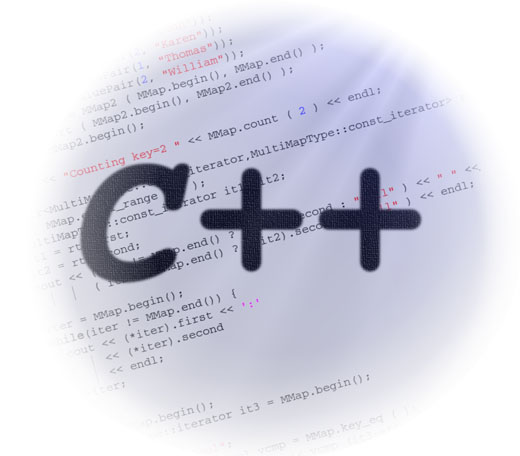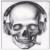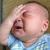C++编译器无法捕捉到的8种错误

CIIS 2019丨CIIS 2019丨倒计时10天丨CIIS 2019 嘉宾阵容公布！>>>C++是一种复杂的编程语言，其中充满了各种微妙的陷阱。在 C++ 中几乎有数不清的方式能把事情搞砸。幸运的是，如今的编译器已经足够智能化了，能够检测出相当多的这类编程陷阱并通过编译错误或编译警告来通知程序员。最 终，如果处理得当的话，任何编译器能检查到的错误都不会是什么大问题，因为它们在编译时会被捕捉到，并在程序真正运行前得到解决。最坏的情况下，一个编译 器能够捕获到的错误只会造成程序员一些时间上的损失，因为他们会寻找解决编译错误的方法并修正。

1）变量未初始化

if (bValue)

// do A  　　else 　　     // do B

int foo ()
{
int nX;
return nX;
}

void increment (int &nValue)
{
++nValue;
}
int foo ()
{
int nX;
increment (nX);
return nX;
}

class Foo
{
private:
int m_nValue;

public:
Foo ();
int GetValue () { return m_bValue; }
};

Foo::Foo ()
{
// Oops, 我们忘记初始化m_nValue 了  　　}
int main ()
{
Foo cFoo;
if (cFoo.GetValue () > 0)
// do something  　　    else 　　        // do something else  　　}

int nValue1, nValue2 = 5;

2）整数除法

C++中的大多数二元操作都要求两个操作数是同一类型。如果操作数的不同类型，其中一个操作数会提升到和另一个操作数相匹配的类型。在 C++ 中，除法操作符可以被看做是 2 个不同的操作：其中一个操作于整数之上，另一个是操作于浮点数之上。如果操作数是浮点数类型，除法操作将返回一个浮点数的值：

float fX = 7;
float fY = 2;
float fValue = fX / fY; // fValue = 3.5

int  nX = 7;
int nY = 2;
int nValue = nX / nY;   //  nValue = 3

float fX = 7. 0;
int nY = 2;
float fValue = fX / nY;
// nY 提升为浮点型，除法操作将返回浮点型值
// fValue = 3.5

int nX = 7;
int nY = 2;
float fValue = nX / nY;  // fValue = 3(不是3.5哦！)

int nX = 7;
int nY = 2;
float fValue = static_cast<float>(nX) / nY;  // fValue = 3.5

z = x / y;  // 这是整数除法还是浮点型除法？

int nZ = nX / nY;     // 整数除法  　　double dZ = dX / dY; // 浮点型除法

3）=  vs  ==

// 如果 nValue 是0，返回1，否则返回 nValue  　　int foo (int nValue)
{
if (nValue = 0)  // 这是个键盘敲击错误 ！  　　        return 1;
else 　　        return nValue;
}

int main ()
{
std::cout << foo (0) << std::endl;
std::cout << foo (1) << std::endl;
std::cout << foo (2) << std::endl;
return 0;
}

0 　　0 　　0

4）混用有符号和无符号数

cout << 1015u;  // 15u 是无符号整数

long double (最高)
double 　　float 　　unsigned long int 　　long int 　　unsigned int 　　int               (最低)

cout << 10u15u;

int nX;
unsigned int nY;
if (nX – nY < 0)
// do something5)  delete  vs  delete []

Foo *pScalar = new Foo;

delete 操作符用来回收由 new 操作符分配的内存空间。如果被销毁的对象是类类型，则该对象的析构函数将被调用。

delete pScalar;

Foo *pArray = new Foo;

delete[] pArray;

6)  复合表达式或函数调用的副作用

x=5

void multiply (int x， int y)
{
using namespace std;
cout << x * y << endl;
}

int main ()
{
int x = 5;
std::cout << multiply (x， ++x);
}

int foo (int x)
{
return x;
}

int main ()
{
int x = 5;
std::cout << foo (x) * foo (++x);
}

if (x == 1 && ++y == 2)
// do something

7）不带breakswitch语句

switch (nValue)
{
case 1: eColor = Color::BLUE;
case 2: eColor = Color::PURPLE;
case 3: eColor = Color::GREEN;
default: eColor = Color::RED;
}

switch (nValue)
{
case 1: eColor = Color::BLUE; break;
case 2: eColor = Color::PURPLE; break;
case 3: eColor = Color::GREEN; break;
default: eColor = Color::RED; break;
}

break 语句终止了 case 语句的执行，因此 eColor 的值将保持为程序员所期望的那样。尽管这是非常基础的 switch/case 逻辑，但很容易因为漏掉一个 break 语句而造成不可避免的“瀑布式”执行流。

8）在构造函数中调用虚函数

class Base
{
private:
int m_nID;

public:
Base ()
{
m_nID = ClassID ();
}

// ClassID 返回一个 class 相关的 ID 号  　　    virtual int ClassID () { return 1;}
int GetID () { return m_nID; }
};

class Derived: public Base
{

public:
Derived ()
{
}
virtual int ClassID () { return 2;}
};

int main ()
{
Derived cDerived;
cout << cDerived.GetID (); // 打印出1，不是2！  　　    return 0;
}

最新评论（14）引用来自“timingbob”的评论

8）在构造函数中调用虚函数

5 delete vs delete []

6 复合表达式或函数调用的副作用

7）不带break的switch语句

1）变量未初始化

1.1 函数中的变量未初始化：和文中说的正相反，多数c++编译器支持警告。
1.2 成员数据的未初始，那完全无法由编译过程来解决，不懂哪门子静态语言的编译器能够解决,以java给个例子：
class XXX
{
private int x;
public getX() { return this->x;}
public setX(int ax) {this->x = ax;}
};

3 = vs ==

4 混用有符和无符s